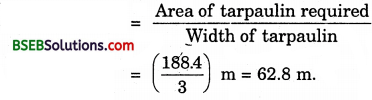# Bihar Board Class 9th Maths Solutions Chapter 13 Surface Areas and Volumes Ex 13.3

Bihar Board Class 9th Maths Solutions Chapter 13 Surface Areas and Volumes Ex 13.3 Textbook Questions and Answers.

## BSEB Bihar Board Class 9th Maths Solutions Chapter 13 Surface Areas and Volumes Ex 13.3Question 1.
Diameter of the base of a cone is 10.5 cm and its slant height is 10 cm. Find its curved surface area.
Solution:
Here r = ($$\frac { 10.5 }{ 2 }$$) cm = 5.25 cm and l = 10.
Curved surface area ofjthe cone = (πrl) cm²
= ($$\frac { 22 }{ 7 }$$ x 5.25 x 10) cm²
= 165 cm².

Question 2.
Find the total surface area of a cone, if its slant height is 21 m and diameter of its base is 24 m.
Solution:
Here r = ($$\frac { 24 }{ 2 }$$) m = 12 m and l = 21 mQuestion 3.
Curved surface area of a cone is 308 cm² and its slant height is 14 cm. Find (i) radius of the base and (ii) total surface area of the cone.
Solution:
(i) Curved surface of a cone = 308 cm²
Slant height, l = 14 cm.
∴ Let r be the radius of the base.
∴ πrl = 308
⇒ $$\frac { 22 }{ 7 }$$ x r x 14 = 308
⇒ r = $$\frac{308 \times 7}{22 \times 14}$$
Thus, the radius of the base = 7 cm

(ii) Total surface area of the cone = πr(l + r) cm²
= $$\frac { 22 }{ 7 }$$ x 7 x (14 x 7) cm²
= (22 x 21) cm²
= 462 cm²Question 4.
A conical tent is 10 m high and the radius of its base is 24 m. Find
(i) slant height of the tent.
(ii) cost of the canvas required to make the tent, if the cost of 1 m² canvas is Rs 70.
Solution:
(i) Here r = 24 m, h = 10 m.
Let l be the slant height of the cone. Then,
l² = h² + r² ⇒ l = $$\sqrt{h^{2}+r^{2}}$$
⇒ l = $$\sqrt{24^{2}+10^{2}}$$ = $$\sqrt{576+100}$$
= $$\sqrt{676}$$ =26 cm

(ii) Canvas required to make the conical tent = Curved surface of the cone
= πrl x($$\frac { 22 }{ 7 }$$ x 24 x 26) cm²
Rate of canvas per 1 m² is Rs 70
∴ Total cost of canvas = Rs ($$\frac { 22 }{ 7 }$$ x 24 x 26 x 70)
= Rs 137280

Question 5.
What length of tarpaulin 3 m wide will be required to make conical tent of height 8 m and base radius 6m? Assume that the extra length of material that will be required for stitching margins and wastage in cutting is approximately 20 cm. (Use n = 3.14)
Solution:
Let r m be the radius, Ambe the height and l m be the slant height of the tent. Then, r = 6m, h = 8 m
⇒ l = $$\sqrt{r^{2}+h^{2}}$$
⇒ l = $$\sqrt{6^{2}+8^{2}}$$
= $$\sqrt{36+64}$$
= $$\sqrt{100}$$ = 10 cm
Area of the canvas used for the tent = curved surface area of the tent = πrl = (3.14 x 6 x 10) m² = 188.4 m²
Now, this area is bought in the form of a rectangle of width 3 m.
∴ Length of tarpaulin requiredThe extra material required for stitching margins and cutting = 20 cm = 0.2 m.
So, the total length of tarpaulin required = (62.8 + 0.2) m = 63 m.Question 6.
The slant height and base diameter of a conical tomb are 25 m and 14 m respectively. Find the cost of white-washing its curved surface at the rate of Rs 210 per 100 m².
Solution:
Here, l = 25 m and r = ($$\frac { 14 }{ 2 }$$) m = 7 m.
Curved surface area = πrl m²
= ($$\frac { 22 }{ 7 }$$ x 25 x 7) m²
= 550 m²
Rate of white-washing is Rs 210 per 100 m²
∴ Cost of white-washing the tomh = Rs ( 550 x $$\frac { 210 }{ 100 }$$ )
= Rs 1155.

Question 7.
A joker’s cap is in the form of a right circular cone of base radius 7 cm and height 24 cm. Find the area of the sheet required to make 10 such caps.
Solution:
Let r cm be the radius, h cm be the height and l cm be the slant height of the joker’s cap. Then, r = 7 cm, h = 24 cmSheet required for 10 such caps = 5500 cm².Question 8.
A bus stop is barricaded from the remaining part of the road, by using 50 hollow cones made of recycled cardboard. Each cone has a base diameter of 40 cm and height 1 m. If the outer side of each of the cones is to be painted and the cost of painting is Rs 12 per m², what will be the cost of painting all these cones ? (Use π = 3.14 and take $$\sqrt{\mathbf{1 . 0 4}}$$ = 1.02)
Solution:
Let r. m be the radius, h m be the height and l m be the slant height of a cone. Then, r = ($$\frac { 40 }{ 2 }$$) cm = 20 cm = 2 m, and h = 1 m
∴ l = $$\sqrt{r^{2}+h^{2}}$$ = $$\sqrt{0.04 + 1}$$
= $$\sqrt{1.04}$$
= 1.02
Curved surface of 1 cone = πrl m²
= ($$\frac { 22 }{ 7 }$$ x .2 x 1.02) m²
Curved surface of such 50 cones
= (50 x $$\frac { 22 }{ 7 }$$ x .2 x 1.02 x 12)
Cost of painting @ Rs 12 per m²
= Rs(50 x $$\frac { 22 }{ 7 }$$ x .2 x 1.02 x 12)
= Rs 384.68 (approx.)